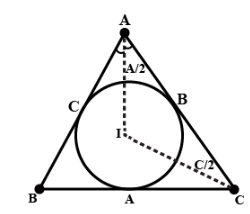QUESTION

# Find the distance of the incentre of the triangle ABC from the point A.A. $4R\sin \dfrac{A}{2}$B. $4R\sin \dfrac{B}{2}\sin \dfrac{C}{2}$C. $4R\cos \dfrac{A}{2}$D. $4R\cos \dfrac{B}{2}\cos \dfrac{C}{2}$

Hint: Incentre is the point in the triangle whose distances from this point to sides are equal. We have to use basic trigonometric angle formulas to solve this problem.

In $\vartriangle AIC,\;\angle AIC = \pi - \left( {\dfrac{{A + C}}{2}} \right)$
$\dfrac{a}{{\operatorname{Sin} a}} = \dfrac{b}{{\operatorname{Sin} b}} = \dfrac{c}{{\operatorname{Sin} c}}$
$\dfrac{{AI}}{{\sin \dfrac{C}{2}}} = \dfrac{b}{{\sin \angle AIC}}$
$\Rightarrow AI = \dfrac{{b\sin \dfrac{C}{2}}}{{\sin \left( {\pi - \dfrac{{A + C}}{2}} \right)}}$
$\Rightarrow AI = \dfrac{{2R\sin Bsin\dfrac{C}{2}}}{{\sin \dfrac{B}{2}}} = \dfrac{{2R\left( {2\sin \dfrac{B}{2}\cos \dfrac{B}{2}} \right)\sin \dfrac{C}{2}}}{{\sin \dfrac{B}{2}}}$
$\Rightarrow AI = 4R\sin \dfrac{B}{2}\sin \dfrac{C}{2}$
$\therefore$ Option B is the correct answer.